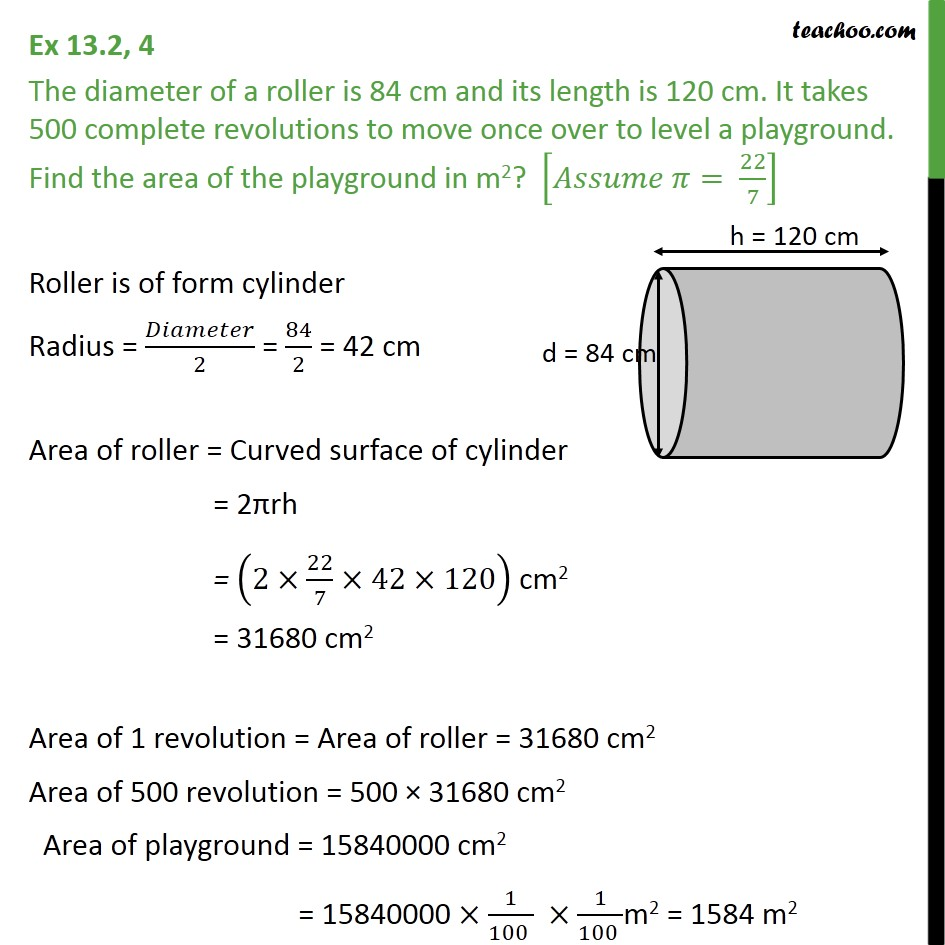Chapter 13 Class 9 Surface Areas and Volumes

Class 9
Important Questions for Exam - Class 9Learn in your speed, with individual attention - Teachoo Maths 1-on-1 Class

### Transcript

Question 4 The diameter of a roller is 84 cm and its length is 120 cm. It takes 500 complete revolutions to move once over to level a playground. Find the area of the playground in m2? [𝐴𝑠𝑠𝑢𝑚𝑒 𝜋= 22/7] Roller is of form cylinder Radius = 𝐷𝑖𝑎𝑚𝑒𝑡𝑒𝑟/2 = 84/2 = 42 cm Area of roller = Curved surface of cylinder = 2πrh = (2×22/7×42×120) cm2 = 31680 cm2 Area of 1 revolution = Area of roller = 31680 cm2 Area of 500 revolution = 500 × 31680 cm2 Area of playground = 15840000 cm2 = 15840000 ×1/(100 ) ×1/(100 )m2 = 1584 m2